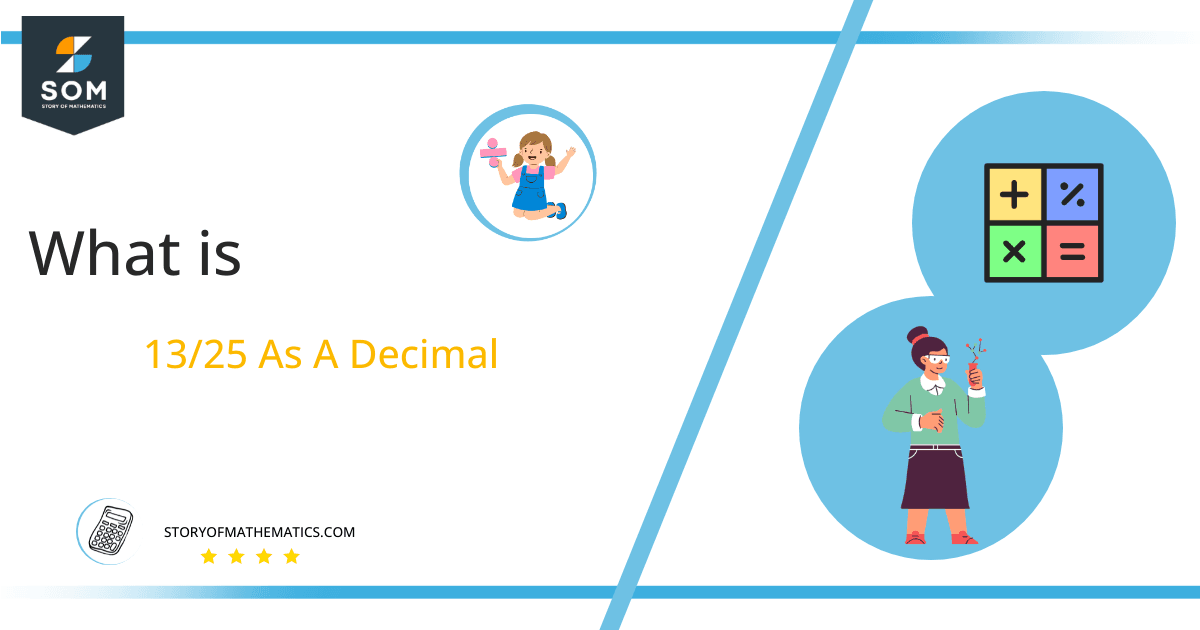# What Is 13/25 as a Decimal + Solution With Free Steps

The fraction 13/25 as a decimal is equal to 0.52.

Fractions are those terms in mathematics which have a denominator and numerator. Fraction has 3 types and these types are proper, improper and mixed fractions. A fraction represents any value or number which has equal parts.

Here, we are interested more in the types of division that results in a Decimal value, as this can be expressed as a Fraction. We see fractions as a way of showing two numbers having the operation of Division between them that result in a value that lies between two Integers.Now, we introduce the method used to solve said fraction to decimal conversion, called Long Division which we will discuss in detail moving forward. So, let’s go through the Solution of fraction 13/25.

## Solution

First, we convert the fraction components i.e., the numerator and the denominator, and transform them into the division constituents i.e., the Dividend and the Divisor respectively.

This can be seen done as follows:

Dividend = 13

Divisor = 25

Now, we introduce the most important quantity in our process of division, this is the Quotient. The value represents the Solution to our division, and can be expressed as having the following relationship with the Division constituents:

Quotient = Dividend $\div$ Divisor = 13 $\div$ 25

This is when we go through the Long Division solution to our problem.Figure 1

## 13/25 Long Division Method

We start solving a problem using the Long Division Method by first taking apart the division’s components and comparing them. As we have 13, and 25 we can see how 13 is Smaller than 25, and to solve this division we require that 13 be Bigger than 25.

This is done by multiplying the dividend by 10 and checking whether it is bigger than the divisor or not. And if it is then we calculate the Multiple of the divisor which is closest to the dividend and subtract it from the Dividend. This produces the Remainder which we then use as the dividend later.

Now, we begin solving for our dividend 13, which after getting multiplied by 10 becomes 130. To indicate this multiplication by 10, we add a decimal “.” to our quotient.

We take this 130 and divide it by 25, this can be seen done as follows:

130 $\div$ 25 $\approx$ 5

Where:

25 x 5 = 125

This will lead to the generation of a Remainder equal to 130 – 125 = 5, now this means we have to repeat the process by Converting the 5 into 50 and solving for that:

50 $\div$ 25 = 2

Where:

25 x 2 = 50

This, therefore, produces another remainder which is equal to 50 – 50 = 0. As the reminder is now zero, we stop here and combine the two pieces of our Quotient to get 0.52, with a final Remainder equal to 0.Images/mathematical drawings are created with GeoGebra.